Courses
Courses for Kids
Free study material
Free LIVE classes
More

# NEET Important Chapter - Work, Energy and Power

Get plus subscription and access unlimited live and recorded courses## Work, Energy and Power: An Important Concept for NEET

Last updated date: 16th Sep 2023
Total views: 141k
Views today: 4.43k

This chapter of work, energy and power  deals with the prior revision of all the concepts we studied in our lower grades to build the connection between the advanced parts of the same. In work, energy and power, we have already studied the formulae which describe physical entities like energy, force and work creating the basics of other derived quantities.

In Physics, work is defined as the scalar product of force and displacement which sometimes is also considered as the work done by a constant force.

W = $\vec{F\cdot\vec{s}}$

Or W=Fs$\cos\theta$

Here F is the force and s is the displacement whereas theta is the angle acting between both of them. This relation helps to understand the various related terms like the angle between force and displacement, and what is the nature of work associated with these two quantities.

Moving further, the chapter brings into light the concepts of work done by variable force, the Work-Energy theorem and some other forms of energy. We have also studied the conservative and non-conservative forces and the stability of equilibrium when different forces act in the system, Gravitational Potential energy motion in the vertical circle, energy related to the spring system, etc.

Now, let us move on to the important concepts and formulae related to NEET exams along with a few solved examples.

### Important Topics of Work, Energy and Power

• Work and its unit

• Work done by a Variable force

• Work Energy Theorem

• Conservative Forces

• The potential energy of spring

• Gravitational Potential Energy

• Vertical Circular Motion

• Elastic Collision

• Inelastic Collision

• Energy and its various types

• Conservation of Mechanical Energy

• Energy and its various types

### Important Concepts of Work, Energy and Power

 Name of the Concept Key Points of the Concept Work and its unit Work as a scalar quantity is defined as the product of force and displacement acting on a body.Work is classified as positive if both the force and displacement are acting in the same direction or the angle between both the physical quantities is less than $90^{\circ}$ and negative when both the quantities are acting in opposite directions to each other or the angle between both the physical quantities is greater than $90^{\circ}$.Similarly, work is considered zero if the force and displacement are perpendicular to each other else the angle between both the physical quantities is equal to $90^{\circ}$.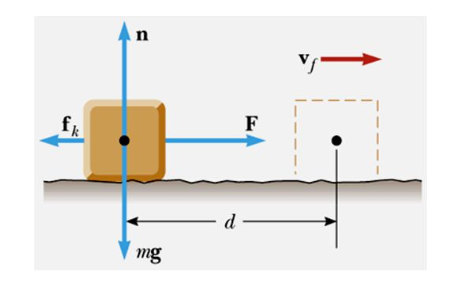The S.I. unit of work is Joule with the dimensional formula as $M^{1}L^{1}T^{-2}$ Work done by a Variable force The formula of Work done by a variable force is calculated as  $\int_{s_{1}}^{s_{2}}\vec{F}(s)\mathrm{d}s$Plotting a graph between force and displacement, we can calculate the work done by variable force by finding the area under the same.Or we can say area under force displacement graph =$\int_{s_{1}}^{s_{2}}\vec{F}(s)\mathrm{d}s$ Energy and its various types Energy is another name of work done. Contrarily, we can say that it refers to the capability of the body to do some kind of work.Kinetic Energy - This energy is related to the virtue of motion of a body, having formula as K.E=$\dfrac{1}{2}mv^{2}$Potential Energy - This energy is related to the change in position of the body in some fieldwith the formula =mgh. Work Energy Theorem This is one of the most important theorems stating that the work done by a net force in displacing a body is the same as the change in kinetic energy of the body.$W_{}=\dfrac{1}{2}mv^{2}-\dfrac{1}{2}mu^{2}= K_{f}-K_{i}$ This formula depicts the entire statement stated above. Conservation of Mechanical Energy This theorem states that the sum of kinetic and potential energy of the body is mechanical energy i.e. E = K+V = $\dfrac{1}{2}mv^{2}$ + mgh.If the force acting on the body is conservative then we can say that mechanical energy of that body is conserved. Conservative Forces If the work done by a body in displacing it from one position to another is independent of the path followed then that force is called Conservative Force.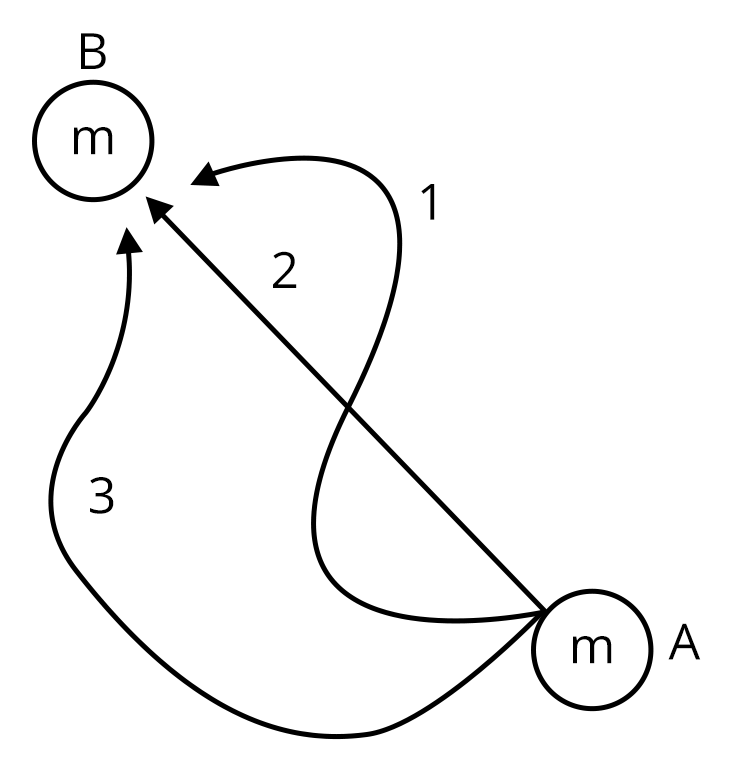Another major characteristic of this force is work done in this case is always zero.Examples of conservative forces are gravitational force, spring force and electric force.If $\triangle\times\bar{F}$=0 then $\bar{F}=-\dfrac{\partial U}{\partial x}$Hence, a conservative force is a negative gradient of potential energy. Other Forms of Energy:-The potential energy of spring This energy is related to the application of force done on a spring system. The external force on the spring produces a restoring force inside the spring due to compression or extension. F=-kxHence, the energy stored in spring system is given by relation :=$\dfrac{1}{2}kx^{2}$Where k is the spring constant and x is the compression or extension in the spring.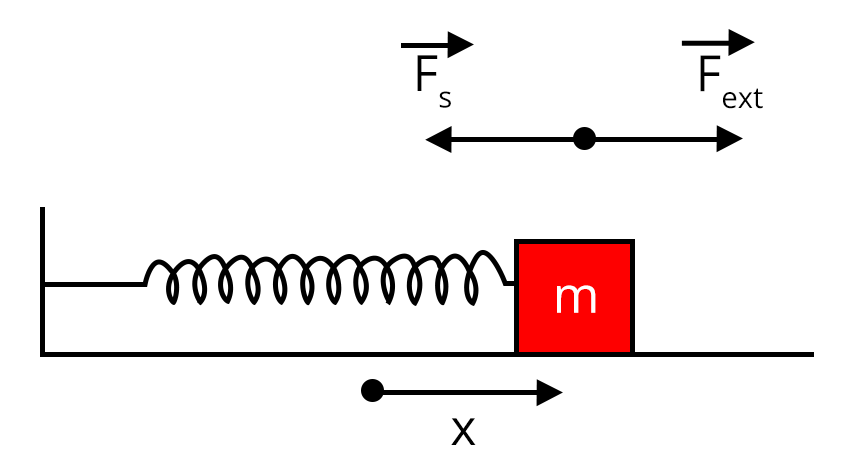Gravitational Potential Energy This is another example of conservative force stated as whenever a body is raised to some height, it acquires some kind of energy due to a change in its position. This energy is called Gravitational Potential Energy.Since the raising of the body is against the direction of earth’s gravity, the force acting here is negative.The work done here is written as W = mgh.U=-$\dfrac{GM}{m}$ for two bodies having mass M and m separated by distance r Vertical Circular Motion Considering a body of mass ‘m’ is tied with a            string (massless) and imparts a velocity at the lowest  point such that it completes the circular trajectory.  A is the lowest point, VA is the velocity imparted to the particle at the lowest point and the length of the string is ‘R’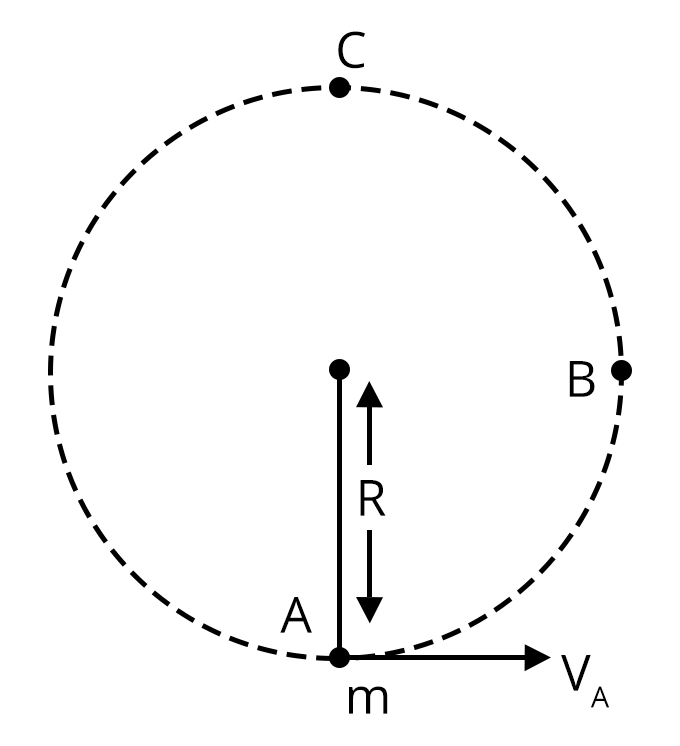Such that tension acting at C(highest point)= $m(v^{2}/r-g)$ whereas tension at lowest point = $(v^{2}/r+g)$.Minimum velocity required by a body to complete circular motion is $\geqslant\sqrt{5rg}$Minimum velocity required by a body to reach at point B = $\geqslant\sqrt{2rg}$ Power Power is defined as a dot product of force and velocity or it is also written as the rate of work done. P= $\dfrac{W}{t}$ or P = $\vec{W\cdot\vec{v}}$The dimensional formula of power is $M^{1}L^{2}T^{-3}$ with the measuring unit as Watt. Collision and its Types Collision is defined as the sticking of two bodies after colliding with each other or if the force exerted by one body  affects the path of another body then the two bodies are said to be colliding.Collision is classified under two categories Elastic and Inelastic. Elastic Collision Those collisions in which the laws of total energy and momentum remain conserved along with conservation of kinetic energy are called elastic collisions.In elastic collision, the relative velocity of separation is equal to relative velocity of approach.Here, the value of coefficient of restitution is 1i.e. e=1 Inelastic Collision Here only the laws of total energy and momentum remain conserved but kinetic energy conservation doesn’t take place.In inelastic collision, the value of the coefficient of restitution lies between 0 and 1.

### List of Important Formulae

 Sl. No Name of the Concept Formula 1. Work W=Fs$\cos\theta$ 2. Work done by a Variable force $\int_{s_{1}}^{s_{2}}\vec{F}(s)\mathrm{d}s$ 3. Energy and its various types Kinetic Energy (K.E)=$\dfrac{1}{2}mv^{2}$Potential Energy =mgh. 4. Conservative Forces $\bar{F}=-\dfrac{\partial U}{\partial x}$ 5. Work- Energy Theorem $W_{}=\dfrac{1}{2}mv^{2}-\dfrac{1}{2}mu^{2}= K_{f}-K_{i}$ 6. Conservation of     Mechanical Energy E = K+V = $\dfrac{1}{2}mv^{2}$ + mgh. 7. Other forms of Energy -The potential energy of spring Work done in spring system=$\dfrac{1}{2}kx^{2}$ 8. Gravitational Potential Energy The Work done here is written as W = mgh.U=-$\dfrac{GM}{m}$ for two bodies having mass M and m separated by distance r 9. Vertical Circular Motion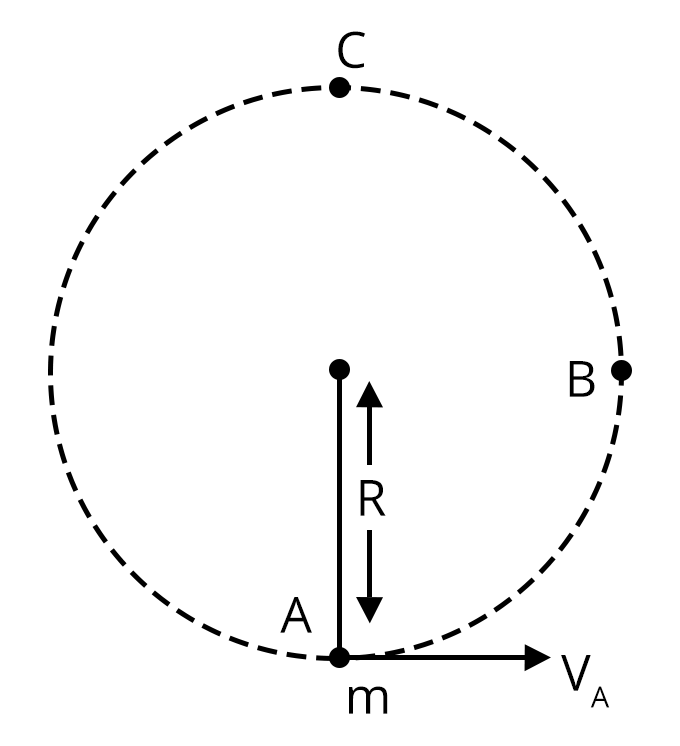Tension acting at C(highest point)= $m(v^{2}/r-g)$ whereas tension at lowest point = $(v^{2}/r+g)$.Minimum velocity required by body to complete circular motion is $\geqslant\sqrt{5rg}$Whereas minimum velocity required by body to reach at point B = $\geqslant\sqrt{2rg}$ 10. Power P=$\dfrac{W}{t}$ or P = $\vec{W\cdot\vec{v}}$ 11. Elastic Collision in one dimension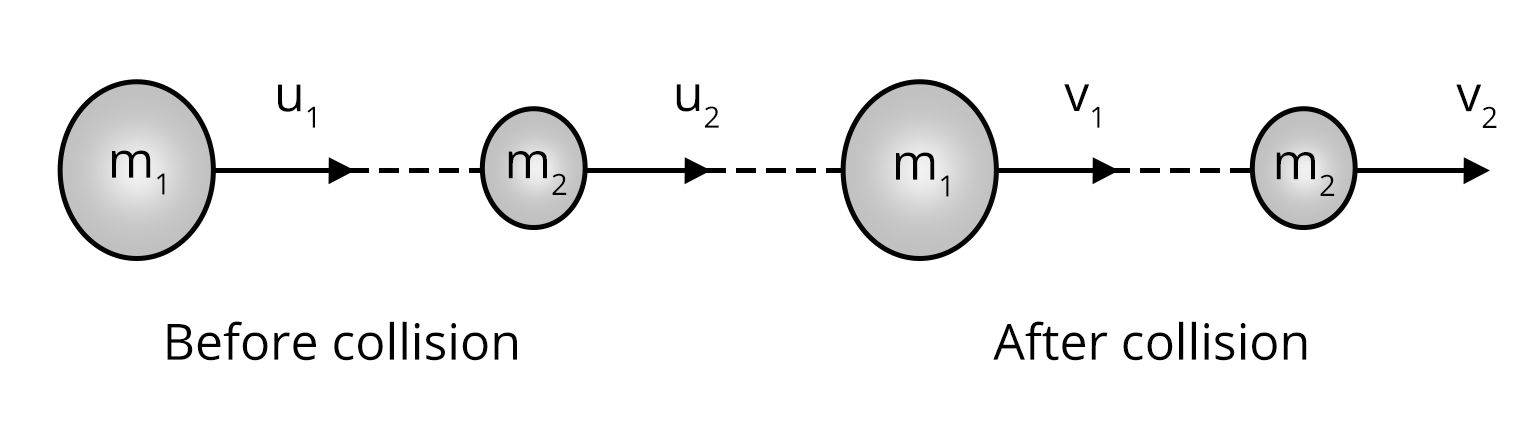Here, the formula of final velocity after collision is$v_{1}$= $(\dfrac{m_{1}-m_{2}}{m_{1}+m_{2}})u_{1}+\dfrac{2m_{2}u_{2}}{m_{1}+m_{2}}$$v_{2}$= $(\dfrac{m_{2}-m_{1}}{m_{1}+m_{2}})u_{2}+\dfrac{2m_{1}u_{1}}{m_{1}+m_{2}}$

### Solved Examples from Work, Energy and Power

1. A porter lifts a suitcase weighing 20kg from the platform and carries it in his hand 2.0m above the platform. Calculate the work done by the porter on the suitcase.

Sol:

Given, $m = 20kg$ , $h = 2m$

$W = -mgh$         (negative sign is due to motion against gravity)

Hence $W = -(20)(10)(2)$ =-400 Joule.

Key Point: Here, only the work done against gravity will act since the kinetic energy of the suitcase is zero.

2. An elevator weighing 500kg is to be lifted up at a constant velocity of 0.20m/s. What would be the minimum horsepower of the motor to be used?

Sol:

Given, m = 500kg, Velocity = 0.20/s

g= 10 m/s2

We have to calculate the power in horsepower.

We know $P= Fv$

Where F is force acting on the elevator. Thus, $F = mg$

Hence, $P = mgv$

=(500)(0.20)(10)

=1000W

And $1hp = 760W$

Therefore, Power in hp = 1000/760 = 1.315hp

Key Point: Here only power’s formula is the main thing that students should remember.

### Previous Year Questions from NEET Paper

1. A force F = 20+10y acts on a particle in y direction where F is in newton and y in metres. The work done by this force to move the particle from y=0 to y=1m is (NEET 2019)

Sol

Given, F = (20+10y) N

We have to calculate the work done from y=0 to y=1m.

Here, the force acting is variable force; hence

W= $\int_{y_{1}}^{y_{2}}Fdy$

= $\int_{y=0}^{y=1}20+10ydy$

=$20y+\left.\dfrac{10^2}{2}\right|_0^1$

=25J

Hence, the work done by this force to move the particle from y=0 to y=1m is 25 J.

Trick: We can solve the question using the formula of power.

2. The work done to raise a mass m from the surface of the earth to a height h, which is equal to the radius of the earth, is:       (NEET 2019)

Sol:

The initial potential energy of the mass at the surface of the earth having radius R is

Ui = $\dfrac{-GMm}{r}$

When mass m is raised to height h, the final energy becomes

Uf = $\dfrac{-GMm}{r+h}$

Hence, the work done here will be the change in kinetic energy = Uf-Ui

= $\dfrac{-GMm}{r+h}$ - $\dfrac{-GMm}{r}$       (here h = r)

=$\dfrac{-GMm}{2r}$ - $\dfrac{-GMm}{r}$

=$\dfrac{-GMm}{2r}$

But g=$\dfrac{GM}{r^{2}}$

Hence, the final work done is $\dfrac{mgR}{2}$

Trick: We can solve the question with the help of the work-energy theorem.

### Practice Questions

1. A cyclist comes to a skidding stop at 10m. During this process, the force on the cycle due to the road is 200N and is directly opposed to the motion. How much work does the road do on the cycle?

Ans -2000J

2. A ball with a velocity of 5 m/s impinges at an angle of 60˚  with the vertical on a smooth horizontal plane. If the coefficient of restitution is 0.5, find the velocity and direction after the impact.

Ans: v = 0.3m/s and the direction will be perpendicular to the surface

### Conclusion

In this article, we have provided all important information regarding the chapter Work, Energy and Power. Some of these include important formulae, concepts, etc. Students should work on more solved examples, sample papers and previous year questions for securing good marks in the NEET exam.

See More## NEET Important Dates

View All Dates
NEET 2023 exam date and revised schedule have been announced by the NTA. NEET 2023 will now be conducted on 7-May-2023, and the exam registration closes on last week of Dec 2022. You can check the complete schedule on our site. Furthermore, you can check NEET 2023 dates for application, admit card, exam, answer key, result, counselling, etc along with other relevant information.
See More
View All Dates## NEET Information

Application Form
Eligibility Criteria
Reservation Policy
NTA has announced the NEET 2023 application form release date on the official website https://neet.nta.nic.in/. NEET 2023 Application Form is available on the official website for online registration. Besides NEET 2023 application form release date, learn about the application process, steps to fill the form, how to submit, exam date sheet etc online. Check our website for more details.## NEET 2023 Study Material

View NEET Syllabus in Detail
View NEET Syllabus in Detail## NEET 2023 Study Material

View all study material for NEET
All
Physics
Chemistry
Biology
See All## NEET Question PapersView all NEET Important Books
Biology
NCERT Book for Class 12 Biology
Physics
NCERT Book for Class 12 Physics
Chemistry
NCERT Book for Class 12 Chemistry
Physics
H. C. Verma Solutions
See All## NEET Mock Tests

View all mock tests
NEET 2023 free online mock test series for exam preparation are available on the Vedantu website for free download. Practising these mock test papers of Physics, Chemistry and Biology prepared by expert teachers at Vedantu will help you to boost your confidence to face the NEET 2023 examination without any worries. The NEET test series for Physics, Chemistry and Biology that is based on the latest syllabus of NEET and also the Previous Year Question Papers.
See More## NEET 2023 Cut Off

NEET Cut Off
NTA is responsible for the release of the NEET 2023 cut off score. The qualifying percentile score might remain the same for different categories. According to the latest trends, the expected cut off mark for NEET 2023 is 50% for general category candidates, 45% for physically challenged candidates, and 40% for candidates from reserved categories. For the general category, NEET qualifying marks for 2022 ranged from 715-117 general category, while for OBC/SC/ST categories, they ranged from 116-93 for OBC, 116-93 for SC and 116-93 for ST category.
See More## NEET 2023 Results

The NEET 2023 result will be published by NTA on https://neet.nta.nic.in/ in the form of a scorecard. The scorecard will include the roll number, application number, candidate's personal details, and the percentile, marks, and rank of the candidate. Only those candidates who achieve the NEET cut-off will be considered qualified for the exam.
See More
Rank List
Counselling
Cutoff
NEET 2023 state rank lists will be released by the state counselling committees for admissions to the {state-quota-percentage} state quota and to all seats in private medical and dental colleges. NEET 2023 state rank lists are based on the marks obtained in entrance exams. Candidates can check the NEET 2023 state rank list on the official website or on our site.## NEET Top Colleges

View all NEET 2023 Top Colleges
Want to know which Engineering colleges in India accept the NEET 2023 scores for admission to Engineering? Find the list of Engineering colleges accepting NEET scores in India, compiled by Vedantu. There are 1622 Colleges that are accepting NEET. Also find more details on Fees, Ranking, Admission, and Placement.
See More## FAQs on NEET Important Chapter - Work, Energy and Power

FAQ

1. What is the weightage of the Work, Energy and Power in NEET?

Nearly 2-3 questions appear in the exam from this chapter covering about 10 marks which makes about 2% of the total marks.

2. What are the key points that need to be practised for solving questions from Work, Energy and Power?

Students should practice the work-energy theorem and vertical circular motion for solving the questions from the  Work, Energy and Power.

3. Are previous year's questions enough for NEET?

To score 600+ in NEET, NCERT (both 11th and 12th) and previous year NEET papers are sufficient. Solving the previous 10 years' question papers for NEET examinations offers a tremendous advantage because 2-3 questions with identical alternatives are guaranteed to be repeated every year.## Notice board

NEET News
NEET Blogs